We can visualize addition using sets: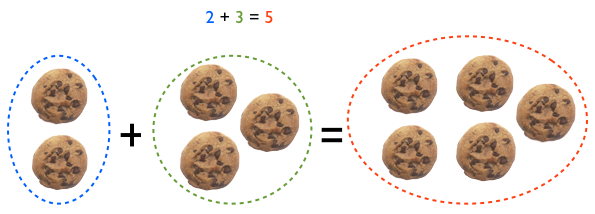or the number line: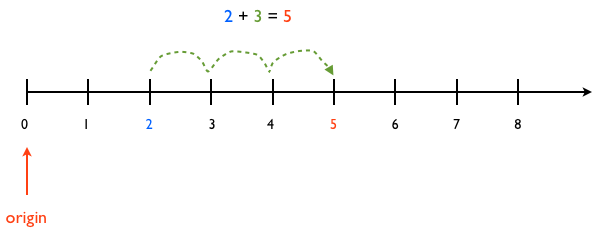Starting at location 2 we advance 3 steps to end in location 5

We can only simplify the addition of unit-less items, or of items that have the same units. For example, we can simplify the addition of unit-less items:
$$5 + 3 = 8$$
and the addition of items with the same units
$$5\mbox{ cookies } + 3\mbox{ cookies } = 8\mbox{ cookies }$$
but we cannot simplify the addition of unit-less items to items with units:
$$5 + 3\mbox{ cookies } = 5 + 3\mbox{ cookies }$$
or the addition of items with different units:
$$5\mbox{ cookies } + 3\mbox{ marbles } = 5\mbox{ cookies } + 3\mbox{ marbles }$$

Although the following two forms describe the same addition process, sometimes it is easier to solve a problem using one form than the other.

$$a + b = x$$

is the same as

\begin{align} a&\\ \underline{+\,\,b}&\\ x& \end{align}

### The Associative law of addition

It says that when we add three numbers, it does not matter which two numbers we add first:

P1. $a + (b + c) = (a + b) + c$

Since regrouping does not affect the result of addition, we usually omit the parentheses but, in reality, the parentheses are implicitly there; it just happens that it does not matter where they are. For example, it does not matter if we mean

$a + b + c = (a + b) + c$

or

$a + b + c = a + (b + c)$

because in both cases the result is the same. From here we get that ,

\begin{align} a + b + c + d & = ((a + b) + c) + d \\ & = (a + (b + c)) + d \\ & = (a + b) + (c + d) \\ & = a + ((b + c) + d) \\ & = a + (b + (c + d)) \\ \end{align}

When adding numbers with multiple digits, it is often useful to use a decimal decomposition, e.g.,

$$1234 = 1000 + 200 + 30 + 4$$

We can use this decomposition to simplify additions, e.g.,

\begin{align} 17 + 34 &= (10 + 7) + (30 + 4) \\ &= (10 + 30) + (7 + 4) \\ &= 40 + 11 \\ &= 51 \\ \end{align}

The U.S. standard addition algorithm adds the digits from right to left, “carrying” sums greater than a digit to the next place value:

\begin{align} &1\,\,\,\,\,\,\,\,\,1\\ &785,927\\ +\,\,&\underline{262,055}\\ 1,&047,982\\ \end{align}

The ‘carry’ is more significant than the number that we are writing under the line; to avoid forgetting to write the ‘carry’, get in the habit of saying, say, 7 + 5 = 12; then write the 12, in that order: first the 1, as a carry, and then the 2, under the line.

Another way to add the numbers is adding by place values; this is longer but avoids errors of forgotten carries.

\begin{align} &\,\,\,\,\,\,\,785,927\\ &\underline{+\,\,262,055}\\ &\,\,\,\,\,\,\,\,\,\,\,\,\,\,\,\,\,\,\,\,\,12\hspace{.2in}⇐ 7 + 5\\ &\,\,\,\,\,\,\,\,\,\,\,\,\,\,\,\,\,\,\,\,\,70\hspace{.2in}⇐ 20 + 50\\ &\,\,\,\,\,\,\,\,\,\,\,\,\,\,\,\,\,\,900\hspace{.2in}⇐ 900 + 0\\ &\,\,\,\,\,\,\,\,\,\,\,\,\,7,\hspace{.48in}⇐ (5 + 2) \times 1,000\\ &\,\,\,\,\,\,\,140,\hspace{.48in}⇐ (80 + 60) \times 1,000\\ &\underline{+\,\,900,\hspace{.45in}}⇐ (700 + 200) \times 1,000\\ &\,1,047,982\\ \end{align}

### Writing sums of sequences

When writing a sum of a sequence we usually use dots, or the symbol for a summation:

\begin{align} \sum_{i = 1}^{10}i &= 1 + 2 + 3 + 4 + 5 + 6 + 7 + 8 + 9 + 10\\ &= 1 + 2 + \dots + 10 \end{align}

The same applies for infinite sums:

$$\sum_{i = 1}^{\infty}i = 1 + 2 + 3 + \dots$$

An easy ways to add sequences of numbers is using Gaussian addition. Say that we want to add the numbers from 1 to 5:

$$1 + 2 + 3 + 4 + 5 = V$$

We can obtain the same result if the write the sequence backwards:

$$5 + 4 + 3 + 2 + 1 = V$$

Now, we pair up the terms of each sum to obtain two times our original desired result V: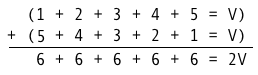Because of the symmetry of the columns, the vertical pairs add up to the same number:

$$6 \times 5 = 2V$$

and we finally solve for V:

$$V = \frac{6 \times 5}{2} = 15$$

In general, the sum of all the numbers from 1 to n is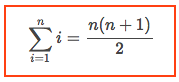We can use Gaussian addition to add many other sequences. For example, let’s add the odd numbers from 20 to 50: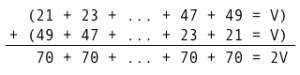There are 15 odd numbers from 20 to 50, so we have that

$$\frac{70 \times 15}{2} = 525$$

Yeah? show me.

### Sets

A set is a collection of things; we can make sets of anything: the set of characters on a page, or the set of children in a room, or the set of animals that fly; each thing in a set is called a member or an element of the set. Sets are useful to group things that share some characteristics, so we can study and understand them better; for example, we can place certain animals into the set of birds, or the set of mammals, or the set of fish. We do the same thing with numbers: to study and understand them better, we divide them in sets, like

Natural numbers (a.k.a. ℕ): we use them to count, like 1, 2, 3,…, etc. There is no universal agreement about whether the natural numbers should include 0 so we have the following more specific sets:

• Whole numbers (a.k.a. non-negative integers) = {0, 1, 2, 3, …}
• Counting numbers (a.k.a. positive integers) = {1, 2, 3 …}

Integer numbers (a.k.a. ℤ, from the german word “Zahlen” that means “to count”): This set contains numbers with no fractions, i.e., the zero, the counting numbers and their negatives:
$$ℤ = {… -3, -2, -1, 0, 1, 2, 3, …}$$

Rational numbers (a.k.a. ℚ, from the word “Quotient”): These numbers are ‘rational’ because they come from ‘ratios’. This set contains all the numbers that are ratios of two integer numbers, and all their corresponding decimals, whether their representation is finite, or periodic infinite. Some examples are:

• 0 = 0/1
• 5 = 5/1
• -1/2 = -0.5 (finite decimal)
• 1/3 = 0.33333.. (infinite periodic decimal)
• 1/7 = 0.14285714285714285… (infinite periodic decimal)

Irrational numbers (a.k.a. ℝ\ℚ, meaning ‘real minus rationals’): This set contains all the numbers that are not the ratio of two integer numbers; all their decimal representations are infinite and non-periodic. Some examples are:

• $\sqrt{2} = 1.4142135623730951…$
• $\sqrt{3} = 1.7320508075688772…$
• $\pi = 3.141592653589793…$
• $e= 2.718281828459045…$
• $(1 + \sqrt{5})/2 = 1.618033988749895…$ a.k.a. the golden ratio

Real numbers (a.k.a. ℝ): This set contains all the rationals and irrationals, i.e., all the numbers in the number line.

In summary we have the following relationship: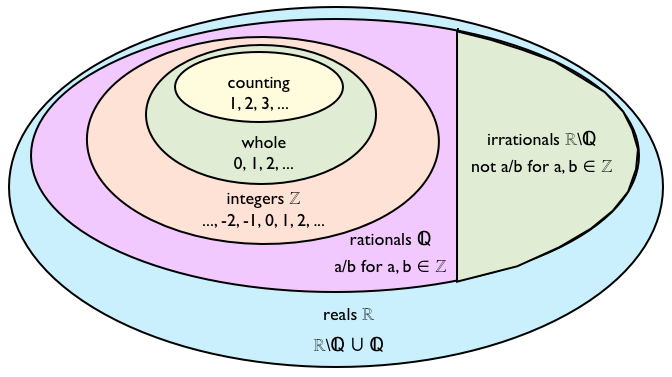### Problems

5 + 3
8 + 9
3 + 8
7 + 5

12 + 34
74 + 15
19 + 83
49 + 72

79 + 145
127 + 63
734 + 125
251 + 84

4. Add using both the U.S. standard and decimal decomposition algorithms

\begin{align} &1,234,567\\ +\,&\underline{2,468,013} \end{align}

5. Find the following sum using both term-by-term addition and Gaussian addition.

$$1 + 2 + \dots + 10 = ?$$

6. Use Gaussian addition to add the odd numbers from 10 to 50

$$11 + 13 + \dots + 49 = ?$$

7. Find $f + g$ for

f = 2x + xy + 4
g = 4x – xy + y + 5

“take all the time you want” problems:

For the following problems use…

P1. $a + (b + c) = (a + b) + c$Associativity

to show that …

8. $((p + q) + r) + s = p + (q + (r + s))$

9. $(p + (q + r)) + s = (p + q) + (r + s)$